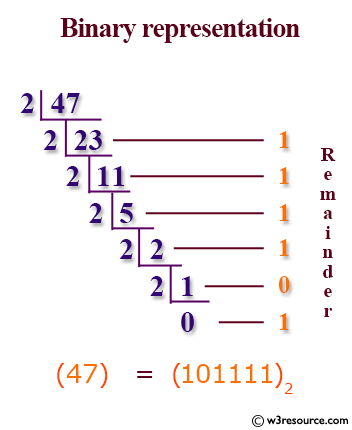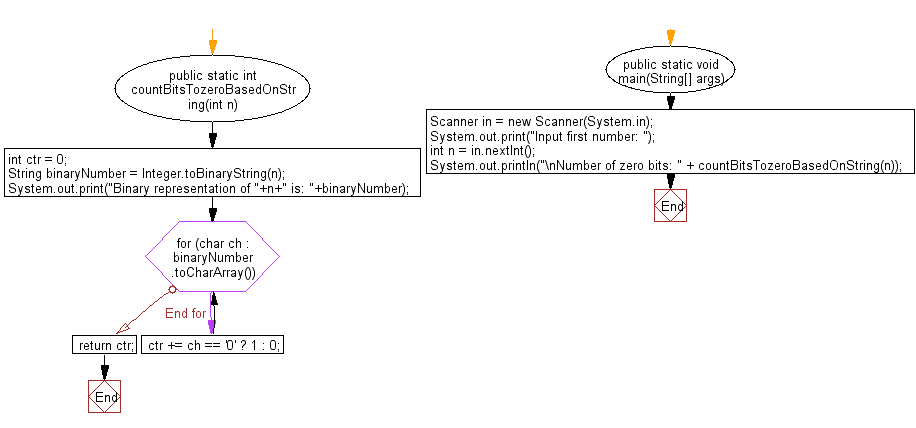﻿ Java programming exercises: Accept an integer and convert it into a binary representation - w3resource# Java Exercises: Accept an integer and convert it into a binary representation

## Java Basic: Exercise-163 with Solution

Write a Java program that will accept an integer and convert it into a binary representation. Now count the number of bits which is equal to zero of the said binary representation.

Pictorial Presentation:Sample Solution:

Java Code:

``````import java.util.Scanner;
public class Solution {
public static int countBitsTozeroBasedOnString(int n) {
int ctr = 0;
String binaryNumber = Integer.toBinaryString(n);
System.out.print("Binary representation of "+n+" is: "+binaryNumber);
for (char ch : binaryNumber.toCharArray()) {
ctr += ch == '0' ? 1 : 0;
}
return ctr;
}

public static void main(String[] args) {
Scanner in = new Scanner(System.in);
System.out.print("Input first number: ");
int n = in.nextInt();
System.out.println("\nNumber of zero bits: " + countBitsTozeroBasedOnString(n));
}
}
```
```

Sample Output:

```Input first number:  25
Binary representation of 25 is: 11001
Number of zero bits: 2s
```

Flowchart:Java Code Editor:

What is the difficulty level of this exercise?

﻿

## Java: Tips of the Day

Parsing dates:

```import java.io.*;
import java.util.*;
import java.text.*;

String s = "2001/09/23 14:39";

SimpleDateFormat formatter = new SimpleDateFormat ("yyyy/MM/dd H:mm");
Date d = formatter.parse(s, new ParsePosition(0));
```